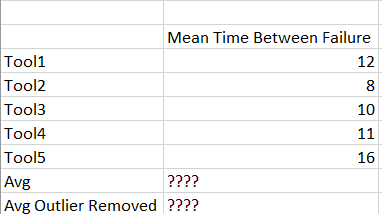# Calculate Average with removed outliers

Hi all,

i have a list of tools and have their mean time between failure in a table.
Therer are items added to the list or removed manually (no automatic filter)
From this list i need the Avg and the Average with outliers removed.

The formular would be:

Calculate Min of the list
Calculate Max of the list

(Sum of whole list - Max - Min ) ( Rows count -2)Hi,

The recommended approach for the calculation of the average of all rows is to use the standard calculation:

This option creates one more column of the average. The good thing is that it automatically adjusts if you manually remove members.

We can find the code behind this calculation and reuse the formula to make one more measure without the min and max values:

The following adjustment will eliminate the rows where the measure is equal to the highest or lowest value:

``````Avg(
Filter(
VisibleRowsSet(),
[Measures].[Mean time between failure]<>
Max(VisibleRowsSet(),[Measures].[Mean time between failure])
AND
[Measures].[Mean time between failure]<>
MIn(VisibleRowsSet(),[Measures].[Mean time between failure])
),
[Measures].[Mean time between failure]
)
``````

Kindly,
Janis, eazyBI support

Thank you so much for your help!!!
It works perfectly.